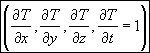The experimental design quality equations as implemented in the applet:

The summation over qualities equation (equivalent, but not identical to Curtis et al., 2002, eq. 6):The product of summation over source qualities equation:where:

a is the partial derivative vectorfor a source-receiver pair

w is the relative weight for each datum (source-receiver pair) as given by the relative amplitude for the source-receiver pair, the relative amplitude is determined by the source-receiver distance and the attenuation relation